Download Presentation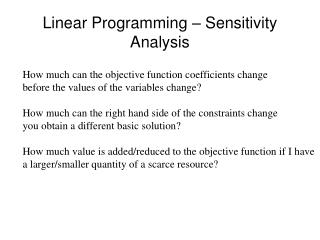Linear Programming – Sensitivity Analysis

# Linear Programming – Sensitivity Analysis - PowerPoint PPT Presentation

Linear Programming – Sensitivity Analysis. How much can the objective function coefficients change before the values of the variables change? How much can the right hand side of the constraints change you obtain a different basic solution?I am the owner, or an agent authorized to act on behalf of the owner, of the copyrighted work described.
Download Presentation## Linear Programming – Sensitivity Analysis

An Image/Link below is provided (as is) to download presentation

Download Policy: Content on the Website is provided to you AS IS for your information and personal use and may not be sold / licensed / shared on other websites without getting consent from its author.While downloading, if for some reason you are not able to download a presentation, the publisher may have deleted the file from their server.

- - - - - - - - - - - - - - - - - - - - - - - - - - E N D - - - - - - - - - - - - - - - - - - - - - - - - - -
Presentation Transcript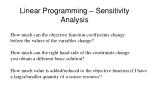Linear Programming – Sensitivity Analysis

How much can the objective function coefficients change

before the values of the variables change?

How much can the right hand side of the constraints change

you obtain a different basic solution?

How much value is added/reduced to the objective function if I have

a larger/smaller quantity of a scarce resource?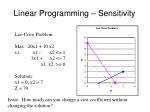Linear Programming – Sensitivity

Leo Coco Problem

Max 20x1 + 10 x2

s.t. x1 - x2 <= 1

3x1 + x2 <= 7

x1, x2 >= 0

Solution:

x1 = 0, x2 = 7

Z = 70

Issue: How much can you change a cost coefficient without changing the solution?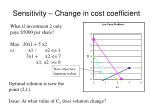Sensitivity – Change in cost coefficient

What if investment 2 only

pays \$5000 per share?

Max 20x1 + 5 x2

s.t. x1 - x2 <= 1

3x1 + x2 <= 7

x1, x2 >= 0

New objective function isobar

Optimal solution is now the point (2,1).

Issue: At what value of C2 does solution change?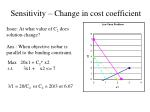Sensitivity – Change in cost coefficient

Issue: At what value of C2 does solution change?

Ans.: When objective isobar is parallel to the binding constraint.

Max 20x1 + C2* x2

s.t. 3x1 + x2 <= 7

3/1 = 20/C2 or C2 = 20/3 or 6.67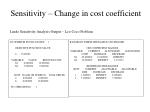Sensitivity – Change in cost coefficient

Lindo Sensitivity Analysis Output – Leo Coco Problem

LP OPTIMUM FOUND AT STEP 1

OBJECTIVE FUNCTION VALUE

1) 70.00000

VARIABLE VALUE REDUCED COST

X1 0.000000 10.000000

X2 7.000000 0.000000

ROW SLACK OR SURPLUS DUAL PRICES

1) 8.000000 0.000000

2) 0.000000 10.000000

NO. ITERATIONS= 1

RANGES IN WHICH THE BASIS IS UNCHANGED:

OBJ COEFFICIENT RANGES

VARIABLE CURRENT ALLOWABLE ALLOWABLE

COEF INCREASE DECREASE

X1 20.000000 10.000000 INFINITY

X2 10.000000 INFINITY 3.333333

RIGHTHAND SIDE RANGES

ROW CURRENT ALLOWABLE ALLOWABLE

RHS INCREASE DECREASE

1 1.000000 INFINITY 8.000000

2 7.000000 INFINITY 7.000000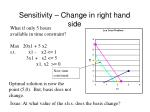Sensitivity – Change in right hand side

What if only 5 hours

available in time constraint?

Max 20x1 + 5 x2

s.t. x1 - x2 <= 1

3x1 + x2 <= 5

x1, x2 >= 0

New time constraint.

Optimal solution is now the point (5,0). But, basis does not change.

Issue: At what value of the r.h.s. does the basis change?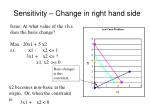Sensitivity – Change in right hand side

Issue: At what value of the r.h.s.

does the basis change?

Max 20x1 + 5 x2

s.t. x1 - x2 <= 1

3x1 + x2 <= ?

x1, x2 >= 0

Basis changes at this constraint.

x2 becomes non-basic at the origin. Or, when the constraint is:

3x1 + x2 < 0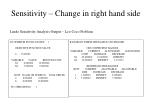Sensitivity – Change in right hand side

Lindo Sensitivity Analysis Output – Leo Coco Problem

LP OPTIMUM FOUND AT STEP 1

OBJECTIVE FUNCTION VALUE

1) 70.00000

VARIABLE VALUE REDUCED COST

X1 0.000000 10.000000

X2 7.000000 0.000000

ROW SLACK OR SURPLUS DUAL PRICES

1) 8.000000 0.000000

2) 0.000000 10.000000

NO. ITERATIONS= 1

RANGES IN WHICH THE BASIS IS UNCHANGED:

OBJ COEFFICIENT RANGES

VARIABLE CURRENT ALLOWABLE ALLOWABLE

COEF INCREASE DECREASE

X1 20.000000 10.000000 INFINITY

X2 10.000000 INFINITY 3.333333

RIGHTHAND SIDE RANGES

ROW CURRENT ALLOWABLE ALLOWABLE

RHS INCREASE DECREASE

1 1.000000 INFINITY 8.000000

2 7.000000 INFINITY 7.000000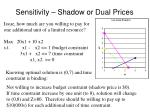Sensitivity – Shadow or Dual Prices

Issue, how much are you willing to pay for one additional unit of a limited resource?

Max 20x1 + 10 x2

s.t. x1 - x2 <= 1 (budget constraint

3x1 + x2 <= 7 (time constraint

x1, x2 >= 0

Knowing optimal solution is (0,7) and time constraint is binding:

Not willing to increase budget constraint (shadow price is \$0).

If time constraint increase by one unit (to 8), solution will change to (0,8) and Z=80. Therefore should be willing to pay up to \$10(000s) for each additional unit of time constraint.Sensitivity – Change in right hand side

Lindo Sensitivity Analysis Output – Leo Coco Problem

LP OPTIMUM FOUND AT STEP 1

OBJECTIVE FUNCTION VALUE

1) 70.00000

VARIABLE VALUE REDUCED COST

X1 0.000000 10.000000

X2 7.000000 0.000000

ROW SLACK OR SURPLUS DUAL PRICES

1) 8.000000 0.000000

2) 0.000000 10.000000

NO. ITERATIONS= 1

RANGES IN WHICH THE BASIS IS UNCHANGED:

OBJ COEFFICIENT RANGES

VARIABLE CURRENT ALLOWABLE ALLOWABLE

COEF INCREASE DECREASE

X1 20.000000 10.000000 INFINITY

X2 10.000000 INFINITY 3.333333

RIGHTHAND SIDE RANGES

ROW CURRENT ALLOWABLE ALLOWABLE

RHS INCREASE DECREASE

1 1.000000 INFINITY 8.000000

2 7.000000 INFINITY 7.000000Linear Programming – Sensitivity Analysis

What if more than one coefficient is changed?:

100% Rule (for objective function coefficients):

if <= 1, the optimal solution will not change,

where is the actual increase (decrease) in the coefficient

and is the maximum allowable increase (decrease) from

the sensitivity analysis.Linear Programming – Sensitivity Analysis

Example obj. function coefficient changesLinear Programming – Sensitivity Analysis

Simultaneous variations in multiple coefficients:

100% Rule (for RHS constants):

if <= 1, the optimal basis and product mix will not change,

where is the actual increase (decrease) in the coefficient

and is the maximum allowable increase (decrease) from

the sensitivity analysis.